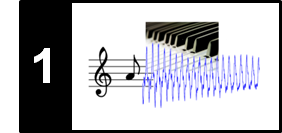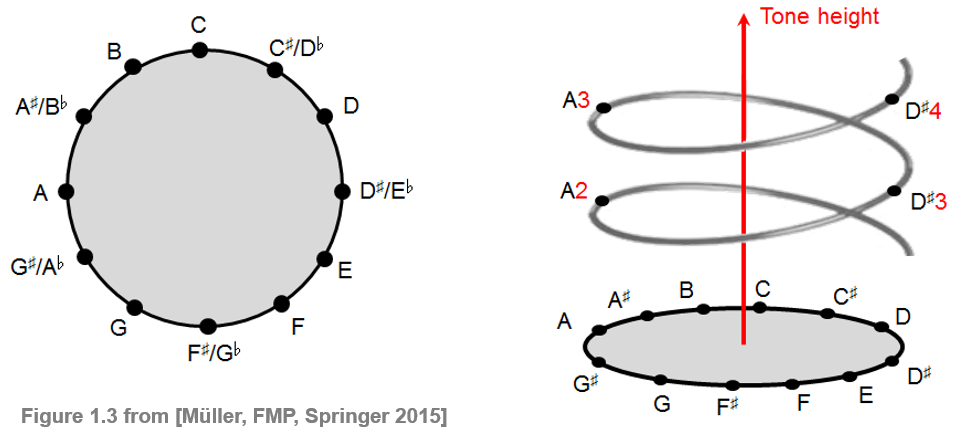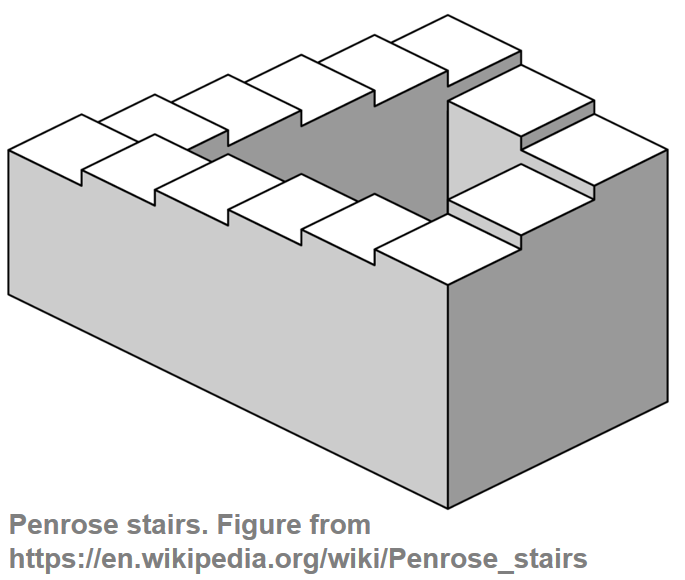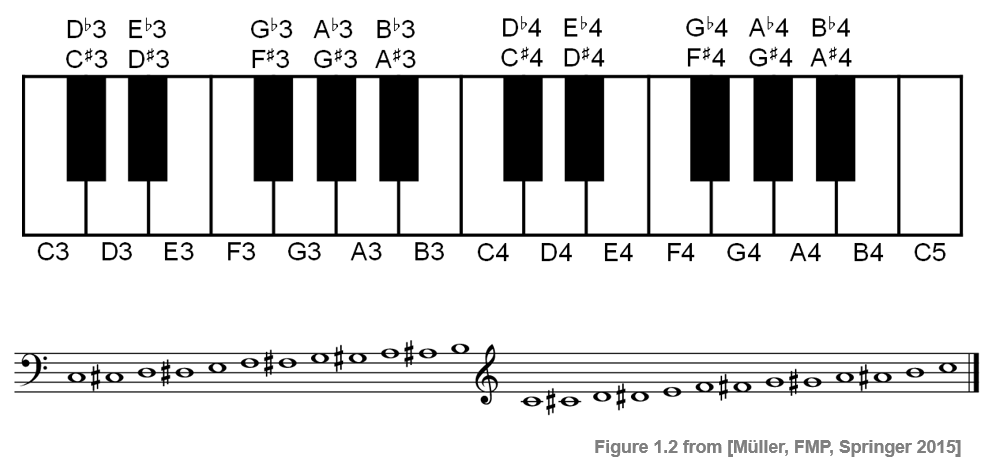# Chroma and Shepard Tones

In this notebook, we discuss the notion of chroma and introduce Shepard's helix of pitch following Section 1.1.1 of [Müller, FMP, Springer 2015]. Furthermore, we introdue a sonfication of chroma using Shepard tones.

## Chroma¶

Ordering all notes of the equal-tempered scale according to their pitches, one obtains an equal-tempered chromatic scale, where all notes of the scale are equally spaced. The term chromatic is derived from the Greek word chroma, meaning color. In the music context, the term chroma closely relates to the twelve different pitch classes. For example, the notes C2 and C5 both have the same chroma value C. In other words, all notes that have the same chroma value belong to the same pitch class.

Notes that belong to the same pitch class (or have the same chroma value) are perceived as similar in a certain way. In contrast, notes that belong to different pitch classes (or have different chroma values) are perceived as dissimilar. This justifies the usage of the term chroma in the sense that notes with different chroma values have a different sound color. The cyclic nature of chroma values is illustrated by the chromatic circle as shown in the following figure. Extending this notion, Shepard's helix of pitch, named after Roger Shepard (* 1929), represents the linear pitch space as a helix wrapped around a cylinder so that octave-related pitches lie along a single vertical line. The projection of the cylinder onto the horizontal plane yields the chromatic circle.## Shepard Tones¶

Shepard's helix of pitch can be sonified using Shepard tones, each being a weighted superposition of sine waves separated by octaves. When playing these tones moving up the chromatic scale, one obtains the auditory illusion of a tone that continuously moves up (similar to the visual illusion of the every ascending Penrose stairs).In the following code example, only sine waves within the human range of hearing (frequencies from approximately 20 to 20000 Hertz) are used. No specific weighting is used, i.e., all sine waves have an amplitude of one. Finally, Shepard tones are generated for a chromatic scale starting with C3 (MIDI pitch 48) and ending wtih C5 (MIDI pitch 72).In :
import numpy as np
import IPython.display as ipd

def generate_shepard_tone(freq=440, dur=0.5, Fs=44100, amp=1):
"""Generate Shepard tone

Notebook: C1/C1S1_ChromaShepard.ipynb

Args:
freq (float): Frequency of Shepard tone (Default value = 440)
dur (float): Duration (in seconds) (Default value = 0.5)
Fs (scalar): Sampling rate (Default value = 44100)
amp (float): Amplitude of generated signal (Default value = 1)

Returns:
x (np.ndarray): Shepard tone
t (np.ndarray): Time axis (in seconds)
"""
N = int(dur * Fs)
t = np.arange(N) / Fs
num_sin = 1
x = np.sin(2 * np.pi * freq * t)
freq_lower = freq / 2
while freq_lower > 20:
num_sin += 1
x = x + np.sin(2 * np.pi * freq_lower * t)
freq_lower = freq_lower / 2
freq_upper = freq * 2
while freq_upper < 20000:
num_sin += 1
x = x + np.sin(2 * np.pi * freq_upper * t)
freq_upper = freq_upper * 2
x = x / num_sin
x = amp * x / np.max(x)
return x, t

def f_pitch(p):
F_A4 = 440
return F_A4 * 2 ** ((p - 69) / 12)

Fs = 44100
dur = 0.5

pitch_start = 48
pitch_end = 72
scale = []
for p in range(pitch_start, pitch_end + 1):
freq = f_pitch(p)
s, t = generate_shepard_tone(freq=freq, dur=dur, Fs=Fs, amp = 0.5)
scale = np.concatenate((scale, s))

ipd.display(ipd.Audio(scale, rate=Fs))